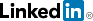cancel
Showing results for
Did you mean:Frequent Visitor

## Inner Filter and Outer Filter not working

hi folks,

I am trying to do a dual filter on a table, running DirectQuery mode, which doesnt seem to work as I hoped - hoping someone here has better ideas.

Example table:

 Outcome date Outcome 1 Outcome Show 4/5/2019 56 700 4/9/2019 78 800 5/23/2019 250 900 7/30/2019 79 1000 7/30/2019 80 1100

what I am trying to do is a table that shows:

 Outcome date Outcome Show 7/30/2019 1100

with this Measure:

Outcome Filter Measure = SUMX(
FILTER(
FILTER(
'Outcome Table',
'Outcome Table'[Outcome Date]=MAX('Outcome Table'[Outcome Date])
),
'Outcome Table'[Outcome 1]=MAX('Outcome Table'[Outcome 1])
),
'Outcome Table'[Outcome Show]*1

Which ends up showing up blank - because the outer filter doesnt seem to work - ie. DAX views them not as sequential filters, but as "AND" filters. So if dont do the second filter, and just filter for MAX Outcome Date, I end up with first row of Max date, showing 1000, while I want to have the table filtered first down to the Latest date availiable, then within that subset, look for the largest Outcome 1 value, then only show resulting row.

Thanks all!

2 REPLIES 2Super User II

Hi @VTB

Try the below.

```Measure =
VAR _date = CALCULATE( MAX( 'Outcome Table'[Outcome date] ), ALL('Outcome Table' ) )
VAR _one = CALCULATE( MAX( 'Outcome Table'[Outcome 1] ), 'Outcome Table'[Outcome date] = _date )
RETURN CALCULATE( SUM( 'Outcome Table'[Outcome Show] ) * 1, 'Outcome Table'[Outcome 1] = _one )```
Best Regards,
Mariusz

If this post helps, then please consider Accepting it as the solution.

Please feel free to connect with me.Frequent Visitor

got the syntax to work, but returns blanks for every row.